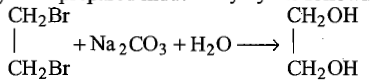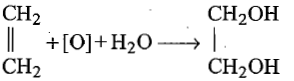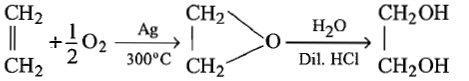The compound which reacts fastest with Lucas reagent is (at room temperature)

(a) butan-1-ol

(b) butan-2-ol

(c) 2-methyl propan-1-ol

(d) 2-methyl propan-2-ol

Concept Questions :-

Alcohols: Preparation, Physical and Chemical Properties
High Yielding Test Series + Question Bank - NEET 2020

Difficulty Level:

The ionisation constant of phenol is higher than that of ethanol because

1. phenoxide ion is bulkier than ethoxide

2. phenoxide ion is stronger base than ethoxide

3. phenoxide ion is stabilised through delocalisation

4. phenoxide ion is less stable than ethoxide

Concept Questions :-

Phenols: Preparation, Properties, Acidic Nature of Phenol
High Yielding Test Series + Question Bank - NEET 2020

Difficulty Level:

The general molecular formula, which represents the homologous series of alkanols is

(a) CnH2nO2        (b) CnH2nO

(c) CnH2n+1O       (d) CnH2n+2O

Concept Questions :-

Alcohols: Preparation, Physical and Chemical Properties
High Yielding Test Series + Question Bank - NEET 2020

Difficulty Level:

$\mathrm{R}-\mathrm{COOH}\to {\mathrm{RCH}}_{2}\mathrm{OH}$. This reduction cannot be done  by:

(a) ${\mathrm{NaBH}}_{4}$                               (b) Na + alcohol

(c) ${\mathrm{LiAlH}}_{4}$                                (d) all of these

Concept Questions :-

Alcohols: Preparation, Physical and Chemical Properties

Difficulty Level:

What mass of isobutylene is obtained from 37 g of tertiary butyl alcohol by heating with 20% H2SO4 at 363 K, if the yield is 65%?

1. 16 g

2. 18.2 g

3. 20 g

4. 22 g

Concept Questions :-

Alcohols: Preparation, Physical and Chemical Properties
High Yielding Test Series + Question Bank - NEET 2020

Difficulty Level:

Glycol is prepared industrially by the following reactions:

(a)(b)(c)(d) none of the above

Concept Questions :-

Alcohols: Preparation, Physical and Chemical Properties
High Yielding Test Series + Question Bank - NEET 2020

Difficulty Level:

How many isomers of C5H11OH will be primary alcohols?

1. 5

2. 4

3. 2

4. 3

Concept Questions :-

Alcohols: Preparation, Physical and Chemical Properties
High Yielding Test Series + Question Bank - NEET 2020

Difficulty Level:

Phenol is less soluble in water. It is due to:

1. non-polar nature of phenol

2. acidic nature of -OH group

3. non-polar hydrocarbon part in it

4. none of the above

Concept Questions :-

Phenols: Preparation, Properties, Acidic Nature of Phenol
High Yielding Test Series + Question Bank - NEET 2020

Difficulty Level:

The decreasing order of boiling points of $1°$,$2°$,$3°$ alcohol is:

1. $1°$>$2°$>$3°$

2. $3°$>$2°$>$1°$

3. $2°$>$1°$>$3°$

4. none of these

Concept Questions :-

Alcohols: Preparation, Physical and Chemical Properties
High Yielding Test Series + Question Bank - NEET 2020

Difficulty Level:

The boiling points of thio-ethers are .... than those of ether.

(a) lesser

(b) equal

(c) higher

(d) none of these

Concept Questions :-

Ethers: Preparation, Physical & Chemical Properties, Uses